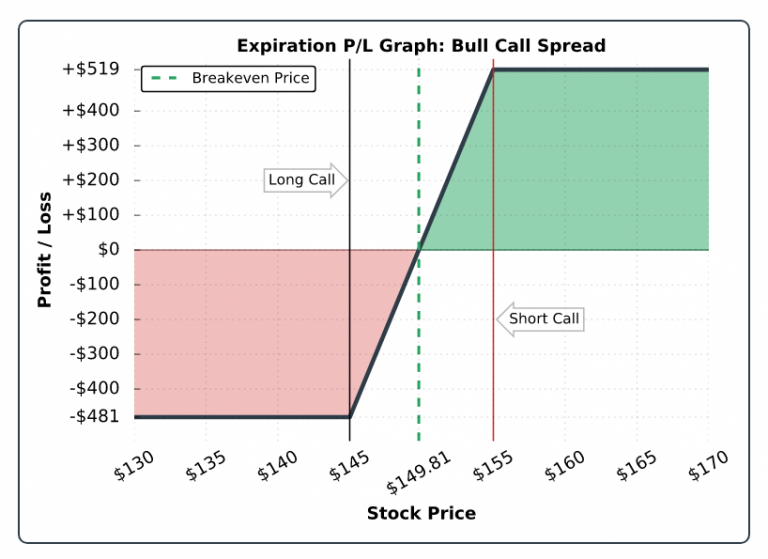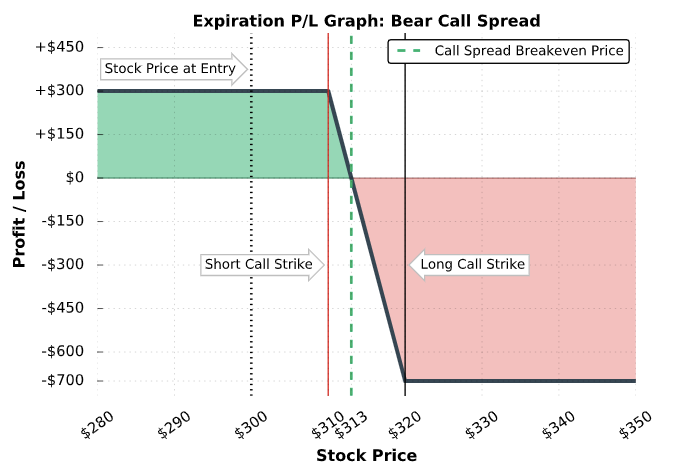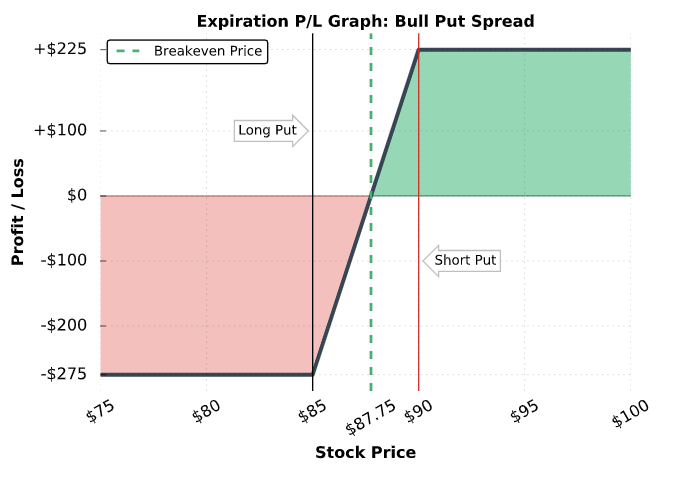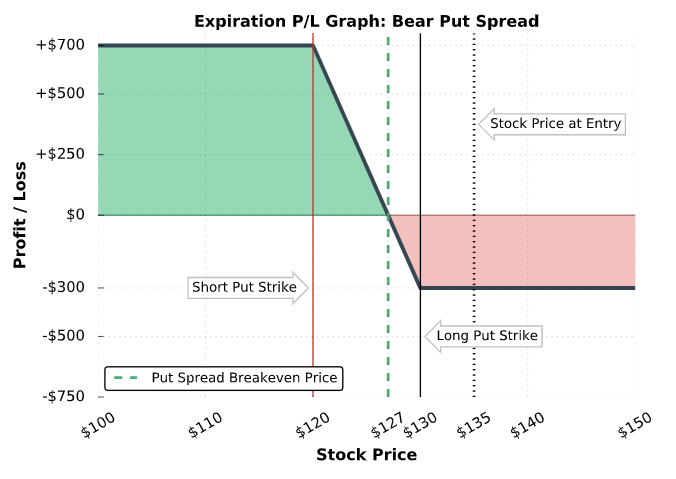# Options Spread Calculator

Created by Arturo Barrantes
Reviewed by Anna Szczepanek, PhD and Steven Wooding
Based on research by
J. Scott Chaput and Louis H. Ederington Option Spread and Combination Trading Portfolio management research (2003)
Last updated: Jul 11, 2022

In the options spread calculator, we continue exploring how to profit with different options strategies:

• Bull call spread option;
• Bull put spread option;
• Bear put spread option, and
• Bear call spread option.

We will start by explaining what an option spread is, and then move on to discussing the concepts of credit spread and debit spread option, and wrap up with some examples.

## What is an option spread? Four option spread strategies

An option spread is a trading strategy where you interact with two call contracts or two put contracts of different strike prices. The difference between the lower strike price and the higher strike price is called option spread.

If you have not checked our excellent call put options calculator yet, we highly recommend you do. You will need the concepts explained there to navigate through this calculator.

For our options spread calculator, we need to clarify the relationship between the buyer and the seller of the call option and the put option:

• When you buy a call option, you are also known as long in the call option. The seller of the call option is known as short. You profit from the price increase. The seller profits if the call expires out of the money because they would keep your premium.

• It is the same for the put options. However, if you are long in the put option, you expect the price to decline so you can sell your market shares at a higher price than the market. Instead, the one who is short in the put earns money (the premium paid by the long put owner) if the option contract expires out of the money.

Investors and traders can negotiate such positions: The long positions can be sold to another investor who also wants to be, for example, long in a call contract. You can also trade the short positions.

Remember that when you are long in an option, you pay a premium (your maximum loss), and when you are short, you receive a premium (your maximum gain). Because stocks do not have an upper limit, your losses can be infinite if you are short in a call option. On the other hand, the stock can go to zero in the case of a put option; thus, if you are short, your losses can be substantial (but limited).

Consequently, when we combine being long and short in calls and puts, we pay a premium in our long positions and receive a premium when short. It is the characteristics of each contract that defines a limited maximum profit and a maximum loss.

This configuration brings two new concepts to our options spread calculator: The net debit spread options and the net credit spread options. You can explore both definitions in the FAQ section of our options spread calculator.

There are four options spread strategies, also known as vertical spread options strategies:

• Bull call spread
• Bear call spread
• Bull put spread
• Bear put spread

We will now discuss them one by one. The formulas explained below are precisely those used by our options spread calculator.

## How to use this options spread calculator?

You have to follow a few steps for using our spectacular options spread calculator:

1. First, choose the calculator mode. Here you have four modes that we can separate into two sections: bullish strategies and bearish strategies:

• Bullish strategies:

• Bull call spread
• Bull put spread
• Bearish strategies:

• Bear call spread
• Bear put spread
2. Carefully enter the data: the price of the call/put option, respective strike price, and amount of acquired contracts.

If your data doesn't obey the conditions of the chosen strategy, the calculator will warn you. 😊

3. Be sure to take time to enjoy the results.

## Bull call spread

The bull call spread is considered a bullish strategy because you profit from the rising price of the underlying asset. Here, you acquire a long call option contract and a short call option contract. Each one with different strike prices. The long call option has to have a lower strike price than the short call option. In other words:

• sp_sc > sp_lc

where:

• sp_sc — Short call strike price; and
• sp_lc — Long call strike price.

Because you are long and short at the same time, the losses you might get from the long call are reduced with the gains of the short call, in the case your asset price ends below the long call strike price. However, if the stock price ends over the short call strike price, the profits from the long call will be capped by the losses of the short call. Here, the profit/loss profile:Let's talk about the formulas that apply at expiration date:

If sc is the short call premium received and lc is the long call premium paid, then the bull call premium spent (ps) satisfies:

• ps = (sc - lc) * n

where n represents the number of spreads we acquire. Then, the maximum loss (ml):

• ml = (sc - lc) * n * 100

The result in both equations will be expressed as negative, reflecting a cash outflow because the premium received by the short call is lower than the premium paid by acquiring the long call. Investors call the cash outflow the debit spread.

Furthermore, the maximum upside profit potential (maxp) and the breakeven price (b) satisfy:

maxp = ((sp_sc - sp_lc) - (lc - sc)) * n * 100

b = sp_lc + (lc - sc)

Finally, the potential profit (pp) at expiration of any stock price (tp) inside the spread:

pp = (tp - sp_lc - (lc - sc)) * n * 100

## Bear call spread

It is a bearish strategy meaning you will profit from a stock fall in price. It includes two call options. Here you will have to sell a call option (a short call) with a lower strike price and to buy a call option (long call) with a higher strike price.

• sp_lc > sp_sc

where:

• sp_lc — Long call strike price; and
• sp_sc — Short call strike price.

Similar to the other bear vertical spread options, you have limited loss potential at the upside and limited profit at the downside.The short call will have a higher premium to be collected, and the long call will have a lower premium to be paid, creating an initial profit called net credit.

If sc is short call premium received and lc is long call premium paid, then the formula for bear call premium earned (pe) reads as:

• pe = (sc - lc) * n

Consequently, the bear call maximum loss (ml), the bear call maximum potential profile (maxp), and the breakeven price (b) will be:

ml = -((sp_lc - sp_sc) - (sc - lc)) * n * 100

maxp = (sc - bec_lc) * n * 100

b = sp_sc + (sc - lc)

As you can see, you earn if the stock remains under the spread. Down below, we are going to cover a bear call spread example. But before, we have to go first to the put spread options sections.

## Bull put spread

In a bull put spread strategy, you profit from a stock increase. It consists of two put options: one of them is a short position, meaning you are selling the put, and the other one is a long position, meaning you are buying the put.

The short put option will have a higher strike price (sp_sp) and a higher premium (sp) you will receive compared to the amount you pay for the long put. On the other hand, the long put will have a lower strike price (sp_lp) and a lower premium to be paid (lp). Consequently, you will earn a premium, making this strategy into a credit spread strategy. So, we have:

• sp_lp < sp_sp

where:

• sp_lp — Long put strike price; and
• sp_sp — Short put strike price.

If we let sp stand for the short put premium received and lp for the long put premium paid, then for the bull put premium earned (pe), we have :

• pe = (sp - lp) * n

The premium earned is also known as net credit. It will also be the maximum profit you could gain from this strategy. The following formulas show the bull put maximum loss (ml), bull put maximum potential profit (maxp), and the breakeven price (b).

ml = -((sp_sp - sp_lp) - (sp - lp)) * n * 100

maxp = (sp - lp) * n * 100

b = sp_sp - (sp - lp)

As you can see, if your stock price ends at expiration below the long put strike price, you incur a maximum loss; whereas, your maximum loss is realized when your stock price ends over the short put strike price at expiration.## Bear put spread

You acquire two options contracts in the bear put spread strategy: one long put with a high strike price and another short put with a lower strike price. Here you profit if the stock falls in price.

• sp_lp > sp_sp

where:

• sp_lp — Long put strike price; and
• sp_sp — Short put strike price.

The long put has a higher premium to pay in the bear put spread, whereas the short put will have a lower premium to collect. Consequently, you will have a debit spread option strategy.

Let sp denote the short put premium received and lp the long put premium paid. Then the bear put premium spent (ps) satisfies:

• ps = -(lp - sp) * n

The following formulas show the bear put maximum loss (ml), bear put maximum potential profit (maxp), and the breakeven price (b). Afterward, we are going to cover a bear put spread example.

ml = -(lp - sp) * n * 100

maxp = ((sp_lp - sp_sp) - (lp - sp)) * n * 100

b = (sp_lp - (lp - sp))

Finally, the profit/loss profile for the bear put spread:The following section will cover examples for the four vertical spread option strategies in our options spread calculator. For the bearish and bullish strategies, we will add a few fundamental recommendations to help you accomplish a better return on investment.

## Vertical spread options examples

#### Bull call spread example

Consider the following setup and five options contracts:

Long call: AMD, strike price = 125 USD, premium paid = 0.77 USD.

Short call: AMD, strike price = 132 USD, premium obtained = 0.19 USD.

You would get:

Net debit spread = (0.19 USD - 0.77 USD) * 5 = -2.9 USD

Max loss = -0.58 USD * 5 * 100 = -290 USD

Maximum profit potential = ((132 USD - 125 USD) - (0.77 USD - 0.19 USD)) * 5 * 100 = 3210 USD

Breakeven price = 125 USD + (0.77 USD - 0.19 USD) = 125.58 USD

If it expires at 130 (you can verify it in our options spread calculator), you would get:

Potential profit at expiration = ((130 USD - 125 USD) - (0.77 USD - 0.19 USD)) * 5 * 100 = 2210 USD

As you can see, the price of the stock has to rise to produce profits. To achieve it, you better keep an eye on its revenue growth and its EPS growth.

#### Bear call spread example

By implementing the following setup and three options contracts:

Long put: ROKU, strike price = 320 USD, premium paid = 9.65 USD.

Short put: ROKU, strike price = 310 USD, premium obtained = 14.35 USD.

We have:

Net credit spread = (14.35 USD - 9.65 USD) = 4.7 USD

Max loss = -(320 USD - 310 USD) - 4.7 USD)* 100 * 3 = -1590 USD

Maximum profit potential = 4.7 USD * 3 * 100 = 1410 USD

Breakeven price = 310 USD + 4.7 USD = 314.7 USD

Note that this is a bearish strategy that profits from a stock fall. Stocks lose price because to several reasons. Some of them are bad coverage over debt and interests.

#### Bull put spread example

Consider the following set up and one options contract:

Long put: AMD, strike price = 115 USD, premium paid = 0.11 USD.

Short put: AMD, strike price = 140 USD, premium obtained = 15.85 USD.

We get:

Net credit spread = (15.85 USD - 0.11 USD) = 15.74 USD

Max loss = (140 USD - 115 USD)- 15.74 USD) * 100 = 926 USD

Maximum profit potential = 15.74 USD * 100 = 1574

Breakeven price = 140 USD - 15.74 USD = 124.26 USD

In this bull put spread example, you can see that you profit if the stock price rise above the breakeven price. For such a price increase, you better keep an eye on its free cash flow growth.

#### Bear put spread example

Let's use the following setup for one contract option.

Long put: AMD, strike price = 130 USD, premium paid = 5 USD.

Short put: AMD, strike price = 120 USD, premium obtained = 2 USD.

The bear put spread strategy will give the following results:

Net debit spread = (2-5) = -3 USD per option

Max loss = - 3 USD * 100 = -300 USD

Maximum profit potential = ((130 USD - 120 USD) - 3) * 100 = 700 USD

Breakeven price = 130 USD - 3 USD = 127

In this bear put spread example, you profit if the stock price falls and ends below the breakeven price. A strong bearish signal of the company's current status is when the operating cash flow starts to decrease.

Options are risky investment vehicles that have made people become millionaires, but also poor. If you are still learning about investing, we recommend you check out our .

## FAQ

### What are the benefits of buying a bull call spread?

In a bull call spread, you benefit from the stock rise in price. However, you also have protection against price fall. Hence, the benefits are:

• Limited loss potential: There is a limit to the amount of money you can lose.
• Reduced cost for entering a long call position: The premium earned by the short call reduces the costs of being long in the call.

### What is a bull call spread?

A bull call spread is a bullish strategy that consists in having two call contracts. First, you buy the long call with a strike price, let's say S1, and immediately afterward, you acquire a short call (here you have the position of the call writer) with a strike price of S2. The long call strike price (S1) shall be lower than the short call strike price (S2). You will earn the maximum profit if the stock price ends above S2.

### What is a credit spread option strategy?

A credit spread option strategy is where the premium received by being short in the contract is more significant than the price paid for being long. The two vertical spread options strategies with net credit spread are the bull put spread and bear call spread.

### What is a debit spread option strategy?

A debit spread option strategy occurs when what you pay for the long position is higher than what you receive for being short. It takes money out of your pocket. The bull call spread and the bear put spread are the two strategies that produce such a situation.

### What are the vertical spread options strategies?

The vertical spread options are setups of contracts that combine two positions at the same time. There are four strategies:

• Bull call spread;
• Bear call spread;
• Bull put spread; and
• Bear put spread.

Each of them contains a long position and a short position of the same type: two calls or two puts, and will have the same expiration day.

Arturo Barrantes
Select calculator mode:
Your target price at expiration
$Amount of options you will trade Purchasing a call with a lower strike price than the short call provides a bullish strategy. The call you buy (long call) Strike price of the call you buy$
$The call you sell (short call) Strike price of the call you sell$
$Bull call estimated returns Net debit spread$
Maximum loss
$Maximum profit$
Breakeven price
$Potential profit$
People also viewed…

### Gmroi

Use this GMROI calculator to determine your gross margin return on inventory.

### Residual income

Our residual income calculator helps you to calculate the economic profit for companies.

### Social Media Time Alternatives

Check what you could have accomplished if you get out of your social media bubble.

### Titration

Use our titration calculator to determine the molarity of your solution.